# GSEB Solutions Class 7 Maths Chapter 15 Visualising Solid Shapes InText Questions

Gujarat Board GSEB Textbook Solutions Class 7 Maths Chapter 15 Visualising Solid Shapes InText Questions and Answers.

## Gujarat Board Textbook Solutions Class 7 Maths Chapter 15 Visualising Solid Shapes InText QuestionsTry These (Page 277)

Question 1.
Match the shape with the name: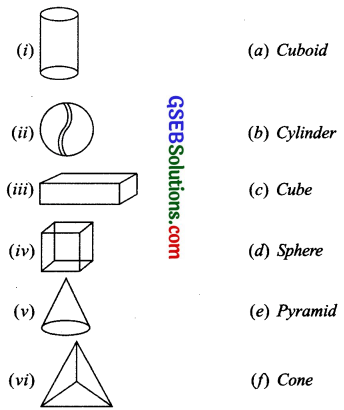Solution:
(i) – (b) Cylinder
(ii) – (d) Sphere
(iii) – (c) Cube
(iv) – (a) Cuboid
(v) – (c) Cube
(v) – (f) Cone
(vi) – (e) PyramidDo This (Page 279)

Question 1.
Complete the following table: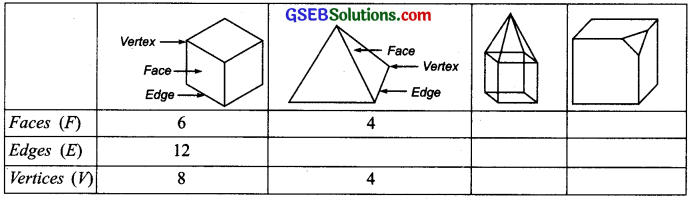Solution:Try These (Page 281)

Question 1.
Here you find four nets. There are two correct nets among them to make a tetrahedron. See if you can work out which nets will make a tetrahedron.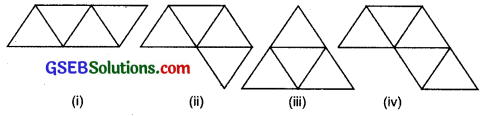Solution:
(i) and (iii) are correct nets to make a tetrahedron.

Try These (Page 286)

Question 1.
Try to guess the number of cubes in the following arrangements.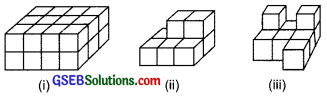Solution:
(i) There are 24 cubes
(ii) There are 8 cubes.
(iii) There are 9 cubes.Try These (Page 287)

Question 1.
Two dice are placed side by side as shown: Can you say what the total would be on the face opposite to(a) 5 + 6
(b) 4 + 3
(Remember that in a die sum of numbers on opposite faces is 7.)
Solution:
(a) The total opposite to face 5 + 6 is 2+1, i.e. 3.
(b) The total opposite to face 4 + 3 is 3 + 4, i.e. 7.

Question 2.
Three cubes each with 2 cm edge are placed side by side to form a cuboid. Try to make an oblique sketch and say what could be its length, breadth and height.Solution:
Length of the cuboid = 2 cm + 2 cm + 2 cm = 6 cm.
Breadth of the cuboid = 2 cm.
Height of the cuboid= 2 cm.Try These (Page 291)

Question 1.
For each solid, the three views (1), (2), (3) are given. Identify for each solid the corresponding top, front and side views.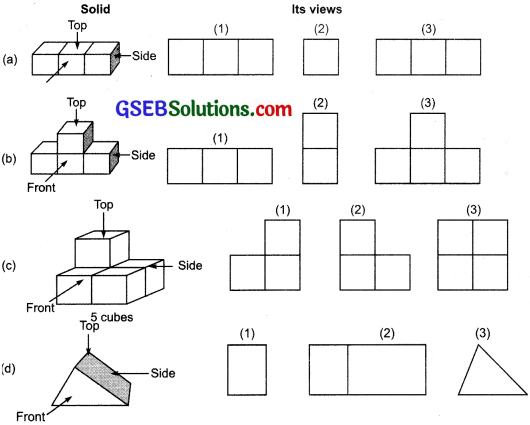Solution:
(a) (1) → Front (2) → Side (3) → Top
(b) (1) → Top (2) → Side (3) → Front
(c) (1) → Side (2) → Front (3) → Top
(d) (1) → Side (2) → Top (3) → FrontQuestion 2.
Draw a view of each solid as seen form the direction indicated by the arrow.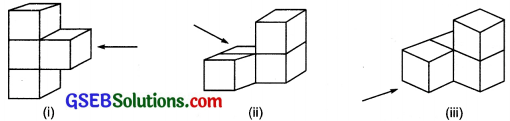Solution: